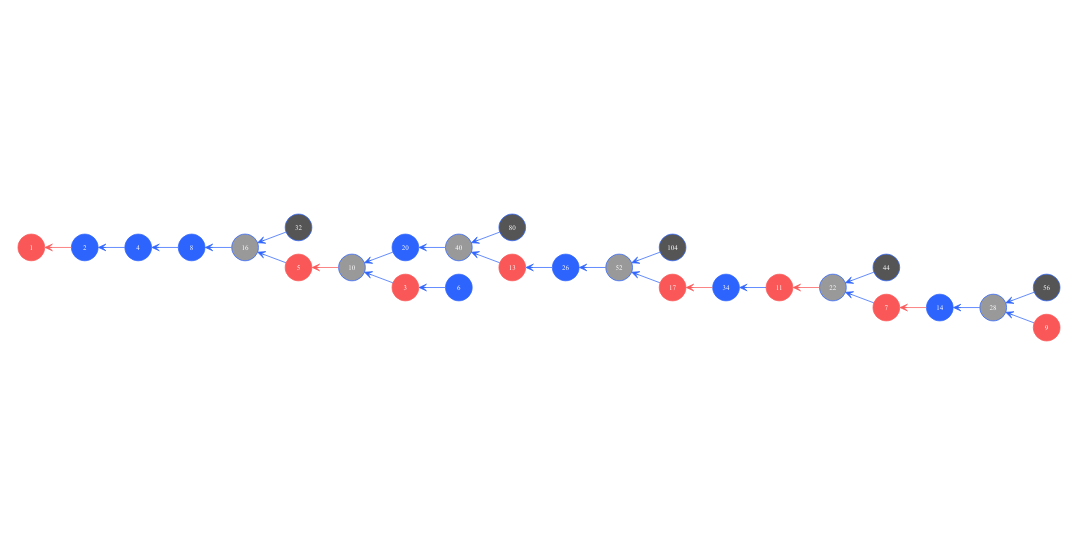Want to share your content on R-bloggers? click here if you have a blog, or here if you don't.

Proof of Collatz sequence convergence.

## Some reminders first

Remember Collatz sequence definitionLet’s divide the Collatz sequence into two independent suites, for analysis convenience.
First suite ω is for numbers: ωn + 1 = 3 ωn + 1
Second suite η is for numbers: ηn + 1 = ηn / 2

## Resolution for numbers from 2 to 11

### resolution graph

First, let’s proceed to an exploratory analysis, and show results as a graph### computation

Doing computation, here is a summary for number 9, that covers all the cases for numbers between 2 and 11.

> cs(9)
 9 28 14 7 22 11 34 17 52 26 13 40 20 10 5 16 8 4 2 1


First important result
Therefore, by calculus, I proved that all the numbers from 2 to 11, provides 1 as final output of Collatz sequence.

## Assumption

Let’s consider the Collatz sequence to be proven up to a given number z. This means that Collatz sequence is proven to be converging for any number m respecting m z.

## Proof for any number e verifying ze2z

As e is an number, then let’s apply η. The result of dividing by two is less than z, and therefore under the assumption stated above, is proven to be converging. Therefore any even number respecting z e 2z is proven to be a valid input for Collatz sequence to be converging.

Now that it is proven for number z e 2z, let’s repeat same reasoning with z’ = 2z. This proves that any number e respecting z’ e 2z’ converges. And so on, proving that any number converges.

Reasoning by recurrence, I got

1. first number that is 2 converges to 1 immediately, just applying η.
2. any number verifying z e 2z, whatever is z converges applying Collatz sequence.

Therefore,

Second important result
All the numbers, converges to 1 as final output of Collatz sequence.

## Proof for any number o

### Direct proof

As o is an number, then let’s apply ω. I already demonstrated that applying ω to and number provides an number. As I have proven here above, that any number brings convergence, therefore, all the numbers also bring convergence.

### Calculus proof

As o is an number, it can be written as ah + bt +u. And as it is an number, then let’s apply ω and η.

starting number cs1 = o = 2p + 1 = ah + bt +u
cs3 = η(ω(s)) = η(ω(2p + 1)) = 3p + 2 = η(ω(ah + bt +u)) = (3ah + 3bt + (3u + 1)) / 2

cs#formulaehundredstensunitcomment
1ah + bt +uabuu ∈ {, , , , }
3(3ah + 3bt + (3u + 1)) / 23a / 2 3b / 2
3b / 2 + 1
3b / 2 + 1
3b / 2 + 2
3b / 2 + 2
(3u + 1) / 2 number
number
number
number
number

Let’s focus on cs3, when it outputs an number. From calculus, shown on previous table, the cases happen when cs1 has a starting unit digit that is amongst , or . As we have previously proven that any number converges, this means that any number finishing with digit , or converges.

Now remain two cases, when cs1 has a starting unit digit that is among or . Here, let’s apply again ω and η as those numbers are . Reusing previous table, the commutation will prove

• cs1 cs3 cs5 number
• cs1 cs3 cs5 number.

Again, as we have previously proven that any number converges, this means that any number finishing with digit or converges.

Third important result
All the numbers, converges to 1 as final output of Collatz sequence.

## Summary

Simply put, I have proven

1. all the numbers from 2 to 11 converges to 1. Proven by calculus
2. all numbers converges to 1. Proven by recurrence
3. all numbers converges to 1. Proven by reusing numbers convergence, based on calculus.

Therefore, proof of Collatz sequence is achieved. Your comments, remarks and feebacks are welcome. Use comment zone to do so.

##### Note

All calculus done using R language.